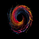7771 views
7771
This is a composite oscillator derived from modified 5/10/20 Chande's Dynamic Momentum Index values.

Volatility is factored in, by design. Look for OB/OS extremes and divergences.

I added the green/red ribbon look to make it easy to read :)

http://www.investopedia.com/terms/d/dyna...

List of my public indicators: http://bit.ly/1LQaPK8
List of my app-store indicators: http://blog.tradingview.com/?p=970

```//
// @author LazyBear
//
// List of my public indicators: http://bit.ly/1LQaPK8
// List of my app-store indicators: http://blog.tradingview.com/?p=970
//
study(title="Chande Composite Momentum Index [LazyBear]", shorttitle="CCMI_LB")
src=input(close, title="Source")
lenSmooth=input(3, title="Composite Smoothing Length")
trigg=input(5, title="Signal Length")
calc_dema(src, length) =>
e1 = ema(src, length)
e2 = ema(e1, length)
2 * e1 - e2

cmo51=sum( iff( src >  src , ( src -  src ) ,0 ) ,5 )
cmo52=sum( iff( src <  src , ( src - src )  ,0 ) ,5 )
cmo5=calc_dema(100 * nz(( cmo51 - cmo52)  /( cmo51+cmo52)),3)
cmo101=sum( iff( src >  src , ( src -  src ) ,0 ) ,10 )
cmo102=sum( iff( src <  src , ( src - src )  ,0 ) ,10 )
cmo10=calc_dema(100 * nz(( cmo101 - cmo102)  /( cmo101+cmo102)),3)
cmo201=sum( iff( src >  src , ( src -  src ) ,0 ) ,20 )
cmo202=sum( iff( src <  src , ( src - src )  ,0 ) ,20 )
cmo20=calc_dema(100 * nz(( cmo201 - cmo202)  /( cmo201+cmo202)),3)
dmi=((stdev(src,5)* cmo5)+(stdev(src,10)* cmo10)+(stdev(src,20)*cmo20))/(stdev(src,5)+stdev(src,10)+stdev(src,20))
e=ema(dmi,lenSmooth), s=sma(dmi,trigg)
hline(70,color=red, title="High2")
ul=hline(30,color=green, title="High1")
hline(0, color=black, title="Mid")
ll=hline(-30,color=green, title="Low1")
hline(-70,color=red, title="Low2")
fill(ul,ll,black, title="MidRegionFill")
duml=plot(e>s?s:e, style=circles, linewidth=0, color=gray, title="Dummy")
cmil=plot(e,title="DynamicIndex",color=blue)
tl=plot(s,title="trigger",color=red)
fill(cmil, duml, color=lime, transp=50, title="PositiveFill")
fill(tl, duml, color=red, transp=50, title="NegativeFill")```Thank you LazyBear for sharing this 'Composite Momentum Index' by Tushar Chande. I noticed a small similarity to William Blau's 'Stochastic Momentum Index', so I created a comparison. Both indicators in my test use the default signal period 5 and a smoothing period of 3:

(I removed your cool color feature for a clearer comparison)

Conclusion: There does not seem to be a clear winner in this comparison, so my recommendation would be to use both indicators to watch the momentum. Here is the 'Stochastic Momentum Index' coded by UCS Gears:

P.S. Both technical analysts are engineers. Tushar Chande holds a Ph.D. in metallurgical engineering from the University of Illinois. And William Blau holds a bachelor's degree in electrical engineering from NYU and a master's in systems engineering and operations research from the University of Pennsylvania.ChartArt
Thanks for the comparison. SMI has TSI in its core (& 5 is the "ergodic" according to Blau), while CMO derives from MOM indirectly too. So, no wonder they all look alike at the end of the day :)

BTW, this is the SMIIO vs the inbuilt SMI vs CMI.LazyBear
Now I get confused. What is the difference between:

Blau's SMII / SMIIO (SMI Ergodic Indicator/Oscillator)
Blau's SMI (Stochastic Momentum Index)

When I set the build-in SMIIO to the same periods as the SMI from UCS then I don't get the same result, The SMI seems to be smoother than the SMIIO.ChartArt
SMIIO is a custom SMI (diff params), as suggested by Anne-Marie Baiynd. More info: